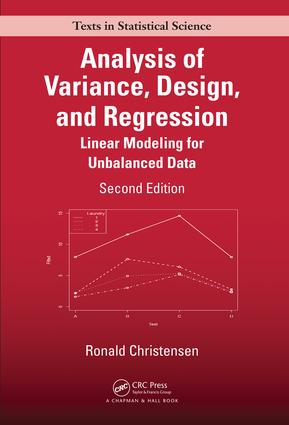Analysis of Variance, Design, and Regression

Linear Modeling for Unbalanced Data, Second Edition, 2nd Edition

Chapman and Hall/CRC

610 pages | 135 B/W Illus.

Purchasing Options:\$ = USD
Hardback: 9781498730143
pub: 2015-12-22
SAVE ~\$24.00
\$120.00
\$96.00
x
eBook (VitalSource) : 9781315370095
pub: 2018-09-03
from \$60.00

FREE Standard Shipping!

Description

Analysis of Variance, Design, and Regression: Linear Modeling for Unbalanced Data, Second Edition presents linear structures for modeling data with an emphasis on how to incorporate specific ideas (hypotheses) about the structure of the data into a linear model for the data. The book carefully analyzes small data sets by using tools that are easily scaled to big data. The tools also apply to small relevant data sets that are extracted from big data.

New to the Second Edition

• Reorganized to focus on unbalanced data
• Reworked balanced analyses using methods for unbalanced data
• Introductions to nonparametric and lasso regression
• Introductions to general additive and generalized additive models
• Examination of homologous factors
• Unbalanced split plot analyses
• Extensions to generalized linear models
• R, Minitab®, and SAS code on the author’s website

The text can be used in a variety of courses, including a yearlong graduate course on regression and ANOVA or a data analysis course for upper-division statistics students and graduate students from other fields. It places a strong emphasis on interpreting the range of computer output encountered when dealing with unbalanced data.

Reviews

Praise for the First Edition:

"… written in a clear and lucid style … an excellent candidate for a beginning level graduate textbook on statistical methods … a useful reference for practitioners."

Zentralblatt für Mathematik

"Being devoted to students mainly, each chapter includes illustrative examples and exercises. The most important thing about this book is that it provides traditional tools for future approaches in the big data domain since, as the author says, the machine learning techniques are directly based on the fundamental statistical methods."

~Marina Gorunescu (Craiova)

Introduction

Probability

Random variables and expectations

Continuous distributions

The binomial distribution

The multinomial distribution

One Sample

Example and introduction

Parametric inference about μ

Prediction intervals

Model testing

Checking normality

Transformations

General Statistical Inference

Model-based testing

Inference on single parameters: assumptions

Parametric tests

Confidence intervals

P values

Validity of tests and confidence intervals

Theory of prediction intervals

Sample size determination and power

The shape of things to come

Two Samples

Two correlated samples: Paired comparisons

Two independent samples with equal variances

Two independent samples with unequal variances

Testing equality of the variances

Contingency Tables

One binomial sample

Two independent binomial samples

One multinomial sample

Two independent multinomial samples

Several independent multinomial samples

Lancaster–Irwin partitioning

Simple Linear Regression

An example

The simple linear regression model

The analysis of variance table

Model-based inference

Parametric inferential procedures

An alternative model

Correlation

Two-sample problems

A multiple regression

Estimation formulae for simple linear regression

Model Checking

Recognizing randomness: Simulated data with zero correlation

Checking assumptions: Residual analysis

Transformations

Lack of Fit and Nonparametric Regression

Polynomial regression

Polynomial regression and leverages

Other basis functions

Partitioning methods

Splines

Fisher’s lack-of-fit test

Multiple Regression: Introduction

Example of inferential procedures

Regression surfaces and prediction

Comparing regression models

Sequential fitting

Reduced models and prediction

Partial correlation coefficients and added variable plots

Collinearity

More on model testing

Additive effects and interaction

Final comment

Diagnostics and Variable Selection

Diagnostics

Best subset model selection

Stepwise model selection

Model selection and case deletion

Lasso regression

Multiple Regression: Matrix Formulation

Random vectors

Matrix formulation of regression models

Least squares estimation of regression parameters

Inferential procedures

Residuals, standardized residuals, and leverage

Principal components regression

One-Way ANOVA

Example

Theory

Regression analysis of ANOVA data

Modeling contrasts

Polynomial regression and one-way ANOVA

Weighted least squares

Multiple Comparison Methods

"Fisher’s" least significant difference method

Scheffé’s method

Studentized range methods

Summary of multiple comparison procedures

Two-Way ANOVA

Unbalanced two-way analysis of variance

Modeling contrasts

Regression modeling

Homologous factors

ACOVA and Interactions

One covariate example

Regression modeling

ACOVA and two-way ANOVA

Near replicate lack-of-fit tests

Multifactor Structures

Unbalanced three-factor analysis of variance

Balanced three-factors

Higher-order structures

Basic Experimental Designs

Experiments and causation

Technical design considerations

Completely randomized designs

Randomized complete block designs

Latin square designs

Balanced incomplete block designs

Youden squares

Analysis of covariance in designed experiments

Discussion of experimental design

Factorial Treatments

Factorial treatment structures

Analysis

Modeling factorials

Interaction in a Latin square

A balanced incomplete block design

Extensions of Latin squares

Dependent Data

The analysis of split-plot designs

A four-factor example

Multivariate analysis of variance

Random effects models

Logistic Regression: Predicting Counts

Models for binomial data

Simple linear logistic regression

Model testing

Fitting logistic models

Binary data

Multiple logistic regression

ANOVA type logit models

Ordered categories

Log-Linear Models: Describing Count Data

Models for two-factor tables

Models for three-factor tables

Estimation and odds ratios

Higher-dimensional tables

Ordered categories

Offsets

Relation to logistic models

Multinomial responses

Logistic discrimination and allocation

Exponential and Gamma Regression: Time-to-Event Data

Exponential regression

Gamma regression

Nonlinear Regression

Introduction and examples

Estimation

Statistical inference

Linearizable models

Appendix A: Matrices and Vectors

Appendix B: Tables

Exercises appear at the end of each chapter.